Search by Topic

Resources tagged with Working systematically similar to Where Can We Visit?:

Filter by: Content type:
Age range:
Challenge level:

There are 127 results

Broad Topics > Using, Applying and Reasoning about Mathematics > Working systematicallyWhere Can We Visit?

Age 11 to 14 Challenge Level:

Charlie and Abi put a counter on 42. They wondered if they could visit all the other numbers on their 1-100 board, moving the counter using just these two operations: x2 and -5. What do you think?Triangles to Tetrahedra

Age 11 to 14 Challenge Level:

Imagine you have an unlimited number of four types of triangle. How many different tetrahedra can you make?Cuboids

Age 11 to 14 Challenge Level:

Find a cuboid (with edges of integer values) that has a surface area of exactly 100 square units. Is there more than one? Can you find them all?Summing Consecutive Numbers

Age 11 to 14 Challenge Level:

15 = 7 + 8 and 10 = 1 + 2 + 3 + 4. Can you say which numbers can be expressed as the sum of two or more consecutive integers?Two and Two

Age 11 to 14 Challenge Level:

How many solutions can you find to this sum? Each of the different letters stands for a different number.Special Numbers

Age 11 to 14 Challenge Level:

My two digit number is special because adding the sum of its digits to the product of its digits gives me my original number. What could my number be?Maths Trails

Age 7 to 14

The NRICH team are always looking for new ways to engage teachers and pupils in problem solving. Here we explain the thinking behind maths trails.9 Weights

Age 11 to 14 Challenge Level:

You have been given nine weights, one of which is slightly heavier than the rest. Can you work out which weight is heavier in just two weighings of the balance?Cayley

Age 11 to 14 Challenge Level:

The letters in the following addition sum represent the digits 1 ... 9. If A=3 and D=2, what number is represented by "CAYLEY"?M, M and M

Age 11 to 14 Challenge Level:

If you are given the mean, median and mode of five positive whole numbers, can you find the numbers?Isosceles Triangles

Age 11 to 14 Challenge Level:

Draw some isosceles triangles with an area of $9$cm$^2$ and a vertex at (20,20). If all the vertices must have whole number coordinates, how many is it possible to draw?Product Sudoku

Age 11 to 14 Challenge Level:

The clues for this Sudoku are the product of the numbers in adjacent squares.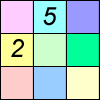Difference Sudoku

Age 14 to 16 Challenge Level:

Use the differences to find the solution to this Sudoku.Reach 100

Age 7 to 14 Challenge Level:

Choose four different digits from 1-9 and put one in each box so that the resulting four two-digit numbers add to a total of 100.Squares in Rectangles

Age 11 to 14 Challenge Level:

A 2 by 3 rectangle contains 8 squares and a 3 by 4 rectangle contains 20 squares. What size rectangle(s) contain(s) exactly 100 squares? Can you find them all?Age 11 to 14 Challenge Level:

How many different symmetrical shapes can you make by shading triangles or squares?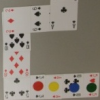Sociable Cards

Age 11 to 14 Challenge Level:

Move your counters through this snake of cards and see how far you can go. Are you surprised by where you end up?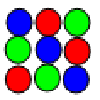Latin Squares

Age 11 to 18

A Latin square of order n is an array of n symbols in which each symbol occurs exactly once in each row and exactly once in each column.Window Frames

Age 5 to 14 Challenge Level:

This task encourages you to investigate the number of edging pieces and panes in different sized windows.Consecutive Negative Numbers

Age 11 to 14 Challenge Level:

Do you notice anything about the solutions when you add and/or subtract consecutive negative numbers?Games Related to Nim

Age 5 to 16

This article for teachers describes several games, found on the site, all of which have a related structure that can be used to develop the skills of strategic planning.More Magic Potting Sheds

Age 11 to 14 Challenge Level:

The number of plants in Mr McGregor's magic potting shed increases overnight. He'd like to put the same number of plants in each of his gardens, planting one garden each day. How can he do it?Age 11 to 14 Challenge Level:

Replace the letters with numbers to make the addition work out correctly. R E A D + T H I S = P A G E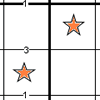Constellation Sudoku

Age 14 to 18 Challenge Level:

Special clue numbers related to the difference between numbers in two adjacent cells and values of the stars in the "constellation" make this a doubly interesting problem.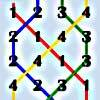Oranges and Lemons, Say the Bells of St Clement's

Age 11 to 14 Challenge Level:

Bellringers have a special way to write down the patterns they ring. Learn about these patterns and draw some of your own.Problem Solving, Using and Applying and Functional Mathematics

Age 5 to 18 Challenge Level:

Problem solving is at the heart of the NRICH site. All the problems give learners opportunities to learn, develop or use mathematical concepts and skills. Read here for more information.More on Mazes

Age 7 to 14

There is a long tradition of creating mazes throughout history and across the world. This article gives details of mazes you can visit and those that you can tackle on paper.Extra Challenges from Madras

Age 11 to 14 Challenge Level:

A few extra challenges set by some young NRICH members.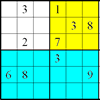Colour Islands Sudoku

Age 11 to 14 Challenge Level:

An extra constraint means this Sudoku requires you to think in diagonals as well as horizontal and vertical lines and boxes of nine.The Great Weights Puzzle

Age 14 to 16 Challenge Level:

You have twelve weights, one of which is different from the rest. Using just 3 weighings, can you identify which weight is the odd one out, and whether it is heavier or lighter than the rest?Weights

Age 11 to 14 Challenge Level:

Different combinations of the weights available allow you to make different totals. Which totals can you make?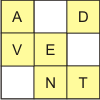Age 11 to 14 Challenge Level:

Rather than using the numbers 1-9, this sudoku uses the nine different letters used to make the words "Advent Calendar".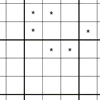Age 11 to 14 Challenge Level:

You need to find the values of the stars before you can apply normal Sudoku rules.Age 7 to 14 Challenge Level:

I added together some of my neighbours' house numbers. Can you explain the patterns I noticed?Star Product Sudoku

Age 11 to 16 Challenge Level:

The puzzle can be solved by finding the values of the unknown digits (all indicated by asterisks) in the squares of the $9\times9$ grid.Football Sum

Age 11 to 14 Challenge Level:

Find the values of the nine letters in the sum: FOOT + BALL = GAMEAdvent Calendar 2011 - Secondary

Age 11 to 18 Challenge Level:

Advent Calendar 2011 - a mathematical activity for each day during the run-up to Christmas.A Long Time at the Till

Age 14 to 18 Challenge Level:

Try to solve this very difficult problem and then study our two suggested solutions. How would you use your knowledge to try to solve variants on the original problem?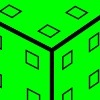Inky Cube

Age 7 to 14 Challenge Level:

This cube has ink on each face which leaves marks on paper as it is rolled. Can you work out what is on each face and the route it has taken?Sticky Numbers

Age 11 to 14 Challenge Level:

Can you arrange the numbers 1 to 17 in a row so that each adjacent pair adds up to a square number?Multiply the Addition Square

Age 11 to 14 Challenge Level:

If you take a three by three square on a 1-10 addition square and multiply the diagonally opposite numbers together, what is the difference between these products. Why?Pair Sums

Age 11 to 14 Challenge Level:

Five numbers added together in pairs produce: 0, 2, 4, 4, 6, 8, 9, 11, 13, 15 What are the five numbers?Number Sandwiches

Age 7 to 14 Challenge Level:

Can you arrange the digits 1, 1, 2, 2, 3 and 3 to make a Number Sandwich?American Billions

Age 11 to 14 Challenge Level:

Play the divisibility game to create numbers in which the first two digits make a number divisible by 2, the first three digits make a number divisible by 3...Number Daisy

Age 11 to 14 Challenge Level:

Can you find six numbers to go in the Daisy from which you can make all the numbers from 1 to a number bigger than 25?Medal Muddle

Age 11 to 14 Challenge Level:

Countries from across the world competed in a sports tournament. Can you devise an efficient strategy to work out the order in which they finished?Multiples Sudoku

Age 11 to 14 Challenge Level:

Each clue in this Sudoku is the product of the two numbers in adjacent cells.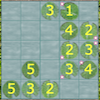Latin Lilies

Age 7 to 16 Challenge Level:

Have a go at this game which has been inspired by the Big Internet Math-Off 2019. Can you gain more columns of lily pads than your opponent?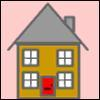Smith and Jones

Age 14 to 16 Challenge Level:

Mr Smith and Mr Jones are two maths teachers. By asking questions, the answers to which may be right or wrong, Mr Jones is able to find the number of the house Mr Smith lives in... Or not!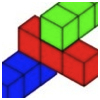Building with Longer Rods

Age 7 to 14 Challenge Level:

A challenging activity focusing on finding all possible ways of stacking rods.